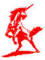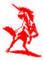Brennan Fitzgerald AssociatesC H A R T E R E D     M A N A G E M E N T     A C C O U N T A N T S H E A LT H C A R E    M A N A G E M E N T    C O N S U L T A N T S I N F O R M A T I O N    T E C H N O L O G Y    C O N S U L T A N T S